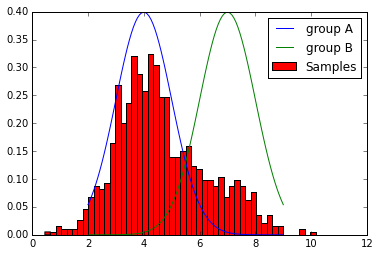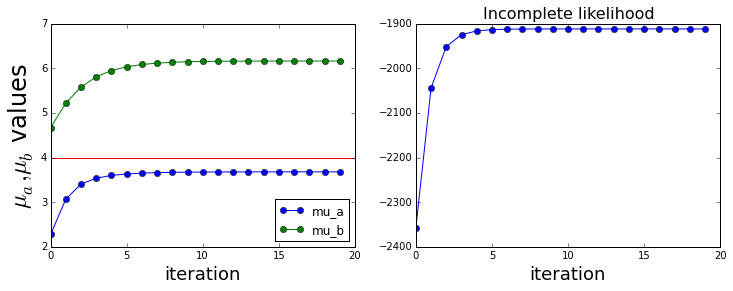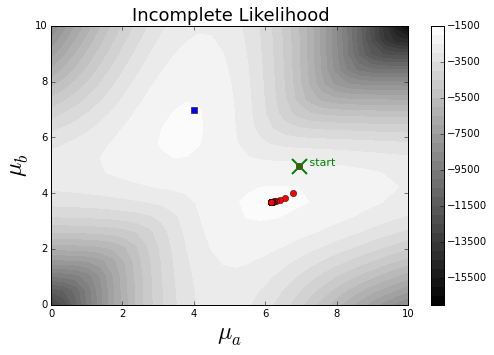## Expectation Maximization¶

Expectation Maximization (EM) is a powerful technique for creating maximum likelihood estimators when the variables are difficult to separate. Here, we set up a Gaussian mixture experiment with two Gaussians and derive the corresponding estimators of their means using EM.

### Experiment: Measuring from Unseen Groups¶

Let's investigate the following experiment:

Suppose we have a population with two distinct groups of individuals with different heights. If we randomly pick an individual from the population, assume we don't know which group the individual is from. So, we measure that individual's height and choose another individual. The goal is to estimate the mean heights of the two distinct groups when we have an unlabeled distribution of heights sampled from both groups.

Group a is normally distributed as

$$\mathcal{N}_a(x) =\mathcal{N}(x; \mu_a,\sigma)$$

and likewise for group b

$$\mathcal{N}_b(x) =\mathcal{N}(x; \mu_b,\sigma)$$

Note that we fix the standard deviation $\sigma$ to be the same for both groups, but the means ($\mu_a,\mu_b$) are different. The problem is to estimate the means given that you can't directly know which group you are picking from.

Then we can write the joint density for this experiment as the following:

$$f_{\mu_a,\mu_b}(x,z)= \frac{1}{2} \mathcal{N}_a(x) ^z \mathcal{N}_b(x) ^{1-z}$$

where $z=1$ if we pick from group a and $z=0$ for group b. Note that the $1/2$ comes from the 50/50 chance of picking either group. Unfortunately, since we do not measure the $z$ variable, we have to integrate it out of our density function to account for this handicap. Thus,

$$f_{\mu_a,\mu_b}(x)= \frac{1}{2} \mathcal{N}_a(x)+\frac{1}{2} \mathcal{N}_b(x)$$

Now, since $n$ trials are independent, we can write out the likelihood:

$$\mathcal{L}(\mu_a,\mu_b|\mathbf{x})= \prod_{i=1}^n f_{\mu_a,\mu_b}(x_i)$$

This is basically notation. We have just substituted everything into $f_{\mu_a,\mu_b}(x)$ under the independent-trials assumption. Recal that the independent trials assumptions means that the joint probability is just the product of the individual probabilities. The idea of maximum likelihood is to maximize this as the function of $\mu_a$ and $\mu_b$ after plugging in all of the $x_i$ data. The problem is we don't know which group we are measuring at each trial so this is trickier than just estimating the parameters for each group separately.

### Simulating the Experiment¶

We need the following code to setup the experiment of randomly a group and then picking an individual from that group.

In :
from __future__ import division
from numpy import array, linspace, random
from scipy.stats import bernoulli, norm
from matplotlib import cm
from matplotlib.pylab import figure, subplots
#random.seed(101) # set random seed for reproducibility
mua_true=4 # we are trying to estimate this from the data
mub_true=7 # we are trying to estimate this from the data
fa=norm(mua_true,1) # distribution for group A
fb=norm(mub_true,1) # distribution for group B
fz=bernoulli(0.25) # each group equally likely

def sample(n=10):
'simulate picking from each group n times'
tmp=fz.rvs(n) # choose n of the coins, A or B
return tmp*(fb.rvs(n))+(1-tmp)*fa.rvs(n) # flip it n times

xs = sample(1000) # generate some samples


Here's a quick look at the density functions of each group and a histogram of the samples

In :
f,ax = subplots()
x = linspace(mua_true-2,mub_true+2,100)
ax.plot(x,fa.pdf(x),label='group A')
ax.plot(x,fb.pdf(x),label='group B')
ax.hist(xs,bins=50,normed=1,label='Samples');
ax.legend(loc=0);Just from looking at this plot, we suspect that we will have to reconcile the samples in the overlap region since these could have come from either group. This is where the Expectation Maximization algorithm enters.

## Expectation maximization¶

The key idea of expectation maximization is that we can somehow pretend we know the unobservable $z$ value and the proceed with the usual maximum likelihood estimation process.

The idea behind expectation-maximization is that we want to use a maximum likelihood estimate (this is the maximization part of the algorithm) after computing the expectation over the missing variable (in this case, $z$).

The following code uses sympy to setup the functions symbolically and convert them to numpy functions that we can quickly evaluate. Because it's easier and more stable to evaluate, we will work with the log of the likelihood function. It is useful to keep track of the incomplete log-likelihood ($\log\mathcal{L}$) since it can be proved that it is monotone increasing and good way to identify coding errors. Recall that this was the likelihood in the case where we integrated out the $z$ variable to reconcile as its absence.

In :
import sympy
from sympy.abc import x, z
from sympy import stats

mu_a,mu_b = sympy.symbols('mu_a,mu_b')
na=stats.Normal( 'x', mu_a,1)
nb=stats.Normal( 'x', mu_b,1)

L=(stats.density(na)(x)+stats.density(nb)(x))/2 # incomplete likelihood function


Next, we need to compute the expectation step. To avoid notational overload, we will just use $\Theta$ to denote the $\mu_b$ and $\mu_a$ parameters and the data $x_i$. This means that the density function of $z$ and $\Theta$ can be written as the following:

$$\mathbb{P}(z,\Theta) = \frac{1}{2} \mathcal{N}_a(\Theta) ^ z \mathcal{N}_b(\Theta) ^ {(1-z)}$$

For the expectation part we have to compute $\mathbb{E}(z|\Theta)$ but since $z\in \lbrace 0,1 \rbrace$, this simplifies easily

$$\mathbb{E}(z|\Theta) = 1 \cdot \mathbb{P}(z=1|\Theta) + 0 \cdot \mathbb{P}(z=0|\Theta) = \mathbb{P}(z=1|\Theta)$$

Now, the only thing left is to find $\mathbb{P}(z=1|\Theta)$ which we can do using Bayes rule:

$$\mathbb{P}(z=1|\Theta) = \frac{ \mathbb{P}(\Theta|z=1)\mathbb{P}(z=1)}{\mathbb{P}(\Theta)}$$

The term in the denominator comes from summing (integrating) out the $z$ items in the full joint density $\mathbb{P}(z,\Theta)$

$$\mathbb{P}(\Theta) = (\mathcal{N}_a(\Theta) + \mathcal{N}_b(\Theta))\frac{1}{2}$$

and since $\mathbb{P}(z=1)=1/2$, we finally obtain

$$\mathbb{E}(z|\Theta) =\mathbb{P}(z=1|\Theta) = \frac{\mathcal{N}_a(\Theta)}{\mathcal{N}_a(\Theta) + \mathcal{N}_b(\Theta)}$$

and which is coded below.

In :
def ez(x,mu_a,mu_b): # expected value of hidden variable
return norm(mu_a).pdf(x) / ( norm(mu_a).pdf(x) + norm(mu_b).pdf(x) )


Now, given we we have this estimate for $z_i$, $\hat{z}_i=\mathbb{E(z|\Theta_i)}$, we can go back and compute the log likelihood estimate of

$$J= \log\prod_{i=1}^n \mathbb{P}(\hat{z}_i,\Theta_i) = \sum_{i=1}^n \hat{z}_i\log \mathcal{N}_a(\Theta_i) +(1-\hat{z}_i)\log \mathcal{N}_b(\Theta_i) +\log(1/2)$$

by maximizing it using basic calculus. The trick is to remember that $\hat{z}_i$ is fixed, so we only have to maximize the $\log$ parts. This leads to

$$\hat{\mu_a} = \frac{\sum_{i=1}^n \hat{z}_i x_i}{\sum_{i=1}^n \hat{z}_i }$$

and for $\mu_b$

$$\hat{\mu_b} = \frac{\sum_{i=1}^n (1-\hat{z}_i) x_i}{\sum_{i=1}^n 1-\hat{z}_i }$$

Now, we finally have the maximization step ( above ) and the expectation step ($\hat{z}_i$) from earlier. We're ready to simulate the algorithm and plot its performance!

In :
Lf=sympy.lambdify((x,mu_a,mu_b), sympy.log(abs(L)),'numpy') # convert to numpy function from sympy

def run():
out, lout = [], []
mu_a_n=random.random() * 10 # initial guess
mu_b_n=random.random() * 10 # initial guess
for i in range(20): # iterations of expectation and maximization
tau=ez(xs,mu_a_n,mu_b_n)                 # expected value of z-variable
lout.append( sum(Lf(xs,mu_a_n,mu_b_n)) ) # save incomplete likelihood value (should be monotone)
out.append((mu_a_n, mu_b_n))             # save of (pa,pb) steps
mu_a_n=( sum(tau*xs) / sum(tau) )        # new maximum likelihood estimate of pa
mu_b_n=( sum((1-tau) * xs) / sum(1-tau) )
return out, lout

out, lout = run()

fig=figure()
fig.set_figwidth(12)
ax.plot(array(out),'o-')
ax.legend(('mu_a','mu_b'),loc=0)
ax.hlines([mua_true,mub_true],0,len(out),['r','g'])
ax.set_xlabel('iteration',fontsize=18)
ax.set_ylabel('$\mu_a,\mu_b$ values',fontsize=24)
ax.plot(array(lout),'o-')
ax.set_xlabel('iteration',fontsize=18)
ax.set_title('Incomplete likelihood',fontsize=16)

Out:
<matplotlib.text.Text at 0xf16bab0>The figure on the left shows the estimates for both $\mu_a$ and $\mu_b$ for each iteration and the figure on the right shows the corresponding incomplete likelihood function. The horizontal lines on the left-figure show the true values we are trying to estimate. Notice the EM algorithm converges very quickly, but because each group is equally likely to be chosen, the algorithm cannot distinguish one from the other. The code below constructs a error surface to see this effect. The incomplete likelihood function is monotone which tells us that we have not made a coding error. We're omitting the proof of this monotonicity.

In :
out, lout = run()
mua_step=linspace(0,10,30)
mub_step=linspace(0,10,20)
fig=figure(figsize=(8,5))
p=ax.contourf(mua_step,mub_step,z,30,cmap=cm.gray)
xa,xb=zip(*out) # unpack the container from the previous block
ax.plot(xa,xb,'ro')                                    # points per iteration in red
ax.plot(mua_true,mub_true,'bs')                        # true values in blue
ax.plot(xa,xb,'gx',ms=15.,mew=2.)                # starting point in green
ax.text(xa,xb,'   start',color='g',fontsize=11.)
ax.set_xlabel('$\mu_a$',fontsize=24)
ax.set_ylabel('$\mu_b$',fontsize=24)
ax.set_title('Incomplete Likelihood',fontsize=18)
fig.colorbar(p);The figure shows the incomplete likelihood function that the algorithm is exploring. Note that the algorithm can get to the maximizer but since the surface has symmetric maxima, it has no way to pick between them and ultimately just picks the one that is closest to the starting point. This is because each group is equally likely to be chosen. I urge you to download this notebook and try different initial points and see where the maximizer winds up.

## Summary¶

Expectation maximization is a powerful algorithm that is especially useful when it is difficult to de-couple the variables involved in a standard maximum likelihood estimation. Note that convergence to the "correct" maxima is not guaranteed, as we observed here. This is even more pronounced when there are more parameters to estimate. There is a nice applet you can use to investigate this effect and a much more detailed mathematical derivation here.

As usual, the IPython notebook corresponding to this post can be found here. I urge you to try these calculations on your own. Try changing the sample size and making the choice between the two groups no longer equal to 1/2 (equally likely).

Note you will need at least sympy version 0.7.2 to run this notebook.